More apps coming soon!

Play or learn. Your choice.

DeLuxe 15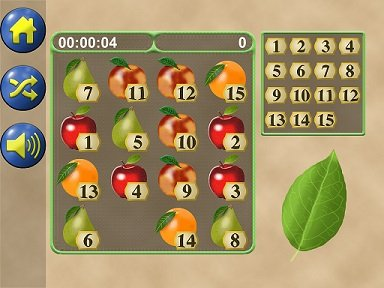Can you solve the 15 puzzle fast enough?

DeLuxe 15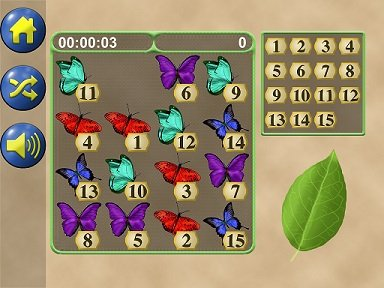Challenge yous friends to beat your best time!

DeLuxe 15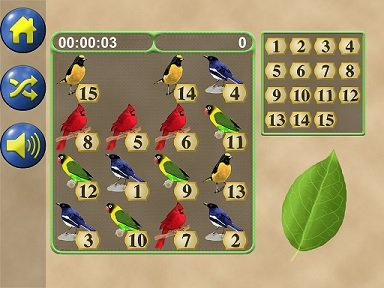Try the different variations!

DeLuxe 15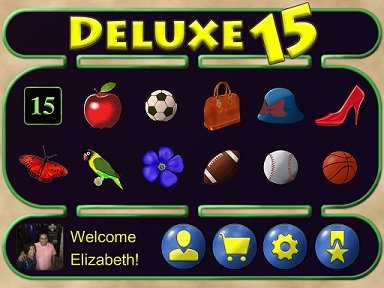Free for you!

Math Fact Games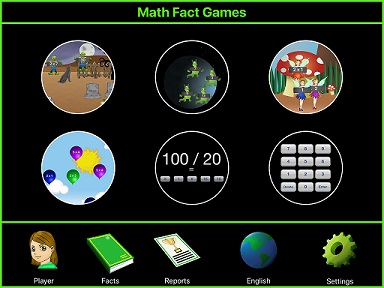Match up problems and answers.

Math Fact Games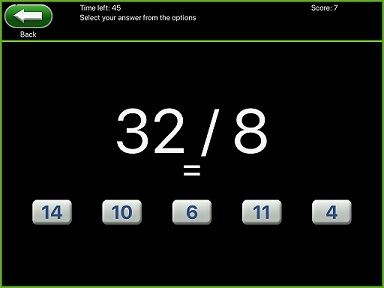Math facts from 1 to 20 with Zombies, astronauts, fairies, balloons.

Math Fact Games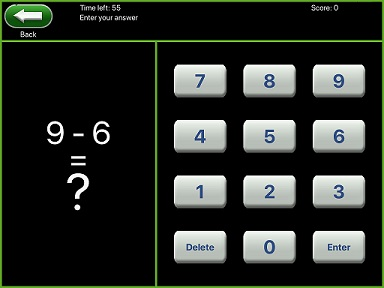Practice Multiplication, division. addition and subtraction.

Math Fact Games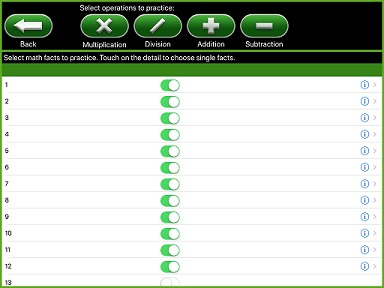All the games in a single App.Math Zombie Facts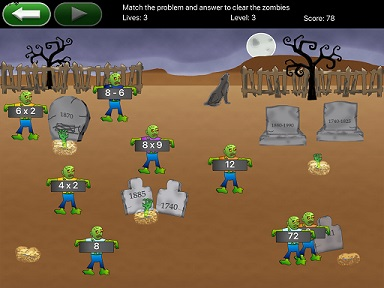Match up problems and answers.

Math Zombie Facts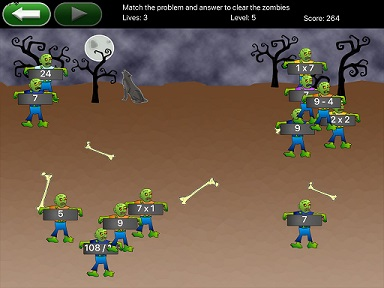Math facts from 1 to 20 with Zombies.

Math Zombie Facts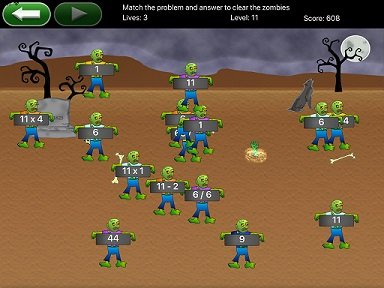Practice Multiplication, division. addition and subtraction.

Math Zombie Facts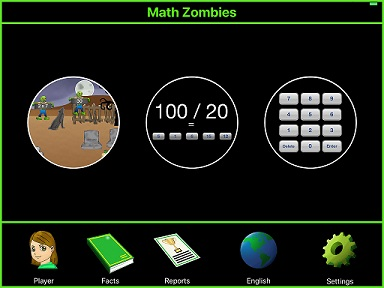Zombies, wolves and tombstones for your fun.

Math Pop Balloons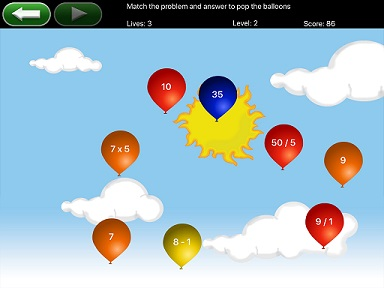Practice Multiplication, division. addition and subtraction.

Math Pop Balloons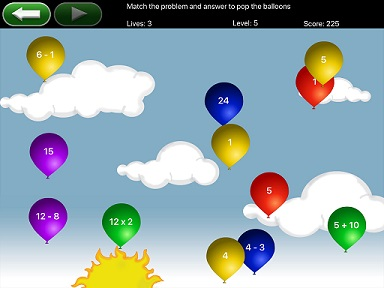Math facts from 1 to 20 with Balloons in a clear sky.

Math Pop Balloons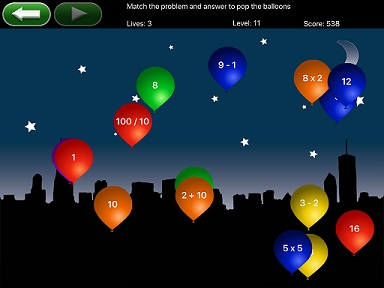Match up problems and answers.

Math Pop Balloons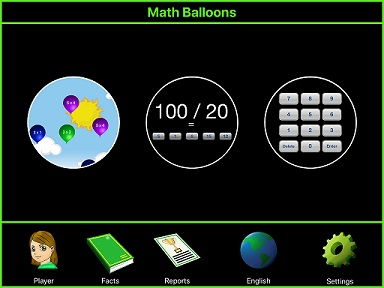Practice with colorfull balloons.Math Space Facts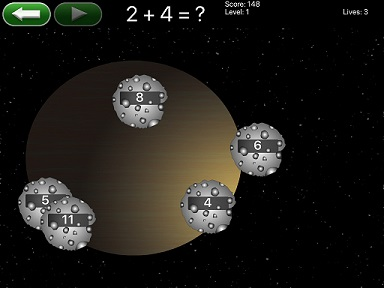Practice Multiplication, division. addition and subtraction.

Math Space Facts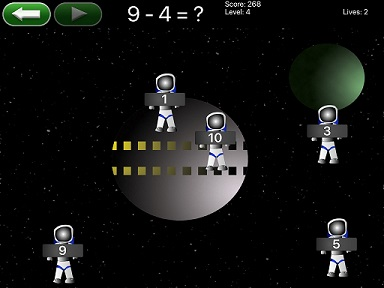Math facts from 1 to 20 with Astronauts in the Space.

Math Space Facts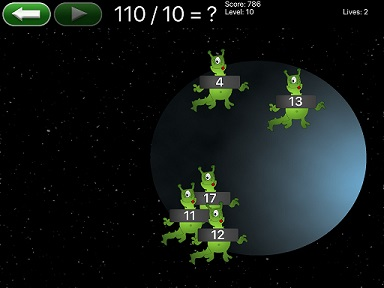Match up problems and answers.

Math Space Facts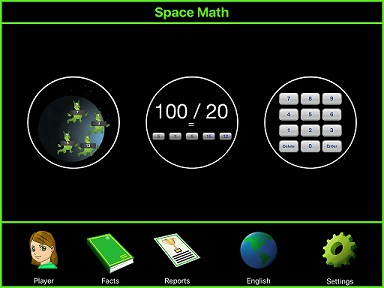Astronauts, aliens and meteorites in the Space.Math Fairy Facts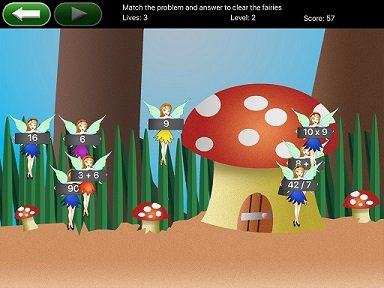Practice Multiplication, division. addition and subtraction.

Math Fairy Facts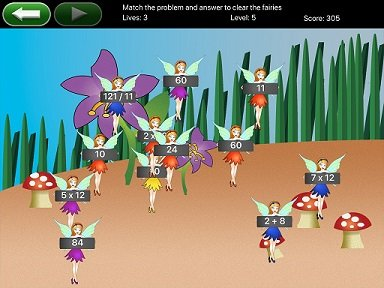Math facts from 1 to 20 with Fairies in an enchanted forrest.

Math Fairy Facts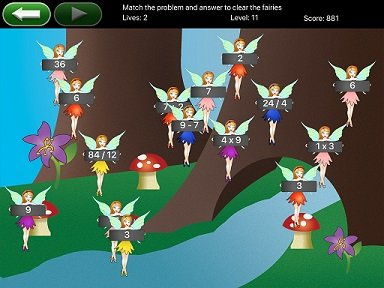Match up problems and answers.

Math Fairy Facts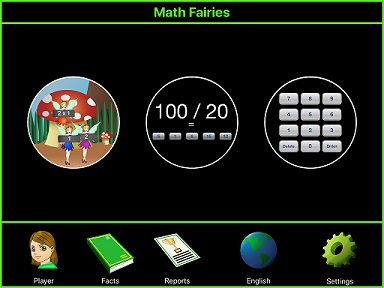Fairies, flowers and trees.